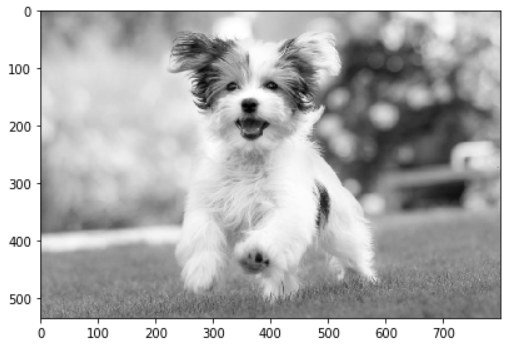# 4 Ways to Convert Image to Grayscale in Python using Skimage, Pillow and OpenCV## Introduction

In this tutorial, we are going to show you multiple ways in which you can convert any image into Grayscale in Python by using different libraries like Skimage, Pillow, and OpenCV. Each of the ways will be shown with examples for easy understanding.

## Different Ways to Convert Image to Grayscale in Python

### Input Image

For all the examples, the below dog image is going to be used as input.### 1. Image Grayscale Conversion with Skimage (Scikit Image) – color.rgb2gray()

Scikit Image or Skimage is a Python based open-source package for various image processing algorithms. Any color image can be converted to grayscale with the help of color.rgb2gray() function of Skimage.

In the below example, the image is read using io.imread() and then it is converted to grayscale with color.rgb2gray() and finally it is displayed with io.imshow()

In :

```from skimage import color
from skimage import io

imgGray = color.rgb2gray(img)

io.imshow(imgGray)```

Out:

<matplotlib.image.AxesImage at 0x7f4161961c90>### 2. Image Grayscale Conversion with Pillow (PIL) – convert()

Pillow is another image processing library of Python which can be used to convert image to grayscale with its img.convert() function.

In this example, the image is read with Image.open() and then it is transformed with convert() by passing ‘L’ as the parameter. The ‘L’ parameter is used to convert the image to grayscale.

In :

```from PIL import Image

img = Image.open('dog.jpg')
imgGray = img.convert('L')
imgGray.show()```

Out:### 3. Image Grayscale Conversion with OpenCV – cv2.imread()

OpenCV is the most popular image processing package out there and there are a couple of ways to transform the image to grayscale. In this first approach, the image can be changed to grayscale while reading the image using cv2.imread() by passing the flag value as 0 along with the image file name.

In:

```import cv2

# Convert to Grayscale

# To display Image
window_name='Grayscale Conversion OpenCV'
cv2.namedWindow(window_name, cv2.WINDOW_NORMAL)
cv2.imshow(window_name,img_gray)
cv2.waitKey(0)
cv2.destroyAllWindows()```

Out:### 4. Image Grayscale Conversion with OpenCV – cv2.cvtColor()

In the next technique, the image can be changed to grayscale with the help of cv2.cvtColor() of OpenCV module which is used for colorspace change.

The parameter to be used with this function for the grayscale is COLOR_BGR2GRAY as we can see in the below example.

In :

```import cv2

# Convert to Grayscale
img_gray = cv2.cvtColor(img,cv2.COLOR_BGR2GRAY)

# To display Image
window_name='Grayscale Conversion OpenCV'
cv2.namedWindow(window_name, cv2.WINDOW_NORMAL)
cv2.imshow(window_name,img_gray)
cv2.waitKey(0)
cv2.destroyAllWindows()```

Out: